# Lazard set

(diff) ← Older revision | Latest revision (diff) | Newer revision → (diff)

A subsetof the free magma, i.e. the free non-associative structure over(cf. also Associative rings and algebras). The elements ofcorrespond to completely bracketed words over(or rooted planar binary trees with leaves labelled by generators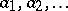; cf. also Binary tree). These are defined recursively as brackets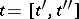where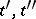are bracketed words of lower weight; bracketed words of weight one correspond to the generators. A subsetis said to be closed, if for each element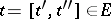one has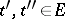. Given two elements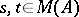, one writes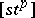to denote the elementConsider treesand subsetsdefined as follows: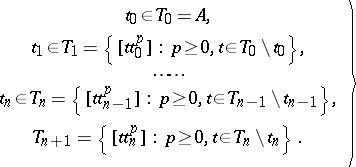(a1)

A Lazard set is a subset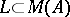such that for any finite, non-empty and closed subsetone has:for some, (a1) holds and, moreover,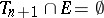.

Lazard sets may be shown to coincide with Hall sets (cf. Hall set). Thus, they give bases of the free Lie algebra over; that is, one may associate a Lie polynomial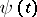to each element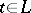of a Lazard set such that the free Lie algebra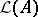(over; cf. Lie algebra, free) is freely generated (as a module over a commutative ring) by the Lie polynomials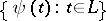. Lazard's elimination process may then be phrased as follows: One has the direct sum decomposition (as a module over a commutative ring):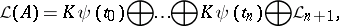where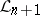is the Lie subalgebra freely generated by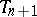.

Lazard sets were introduced by X. Viennot [a1] in order to unify combinatorial constructions of bases of the free Lie algebra. The Lyndon basis (see Lyndon word) was thought to be of a different nature from the one considered by M. Hall [a2], and generalizations of it were proposed by many authors. Viennot gave a unifying framework for all these constructions. One may present Lazard sets in terms of words, rather than trees in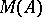. It can then be shown that a unique tree structure is attached to every word of a Lazard set. Moreover, a Lazard set of words is totally ordered, as is a Lazard set of trees, and it is a complete factorization of the free monoid. That is, every word is a unique non-increasing product of Lazard words. This result makes explicit the link between bases of free Lie algebras and complete factorizations of free monoids.